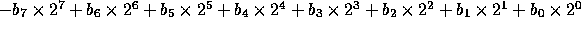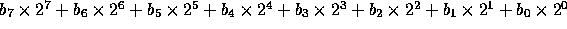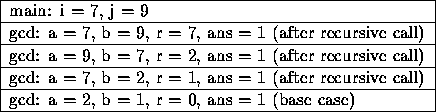# Midterm 1 Solution

CS18

1. Short Answer Problems. Each of the following problems is worth 19 points.

1. What four requirements must be met before a recursive solution to a problem is attempted?
1. Problem must decompose into sub-problems.
2. Base case(s) must exist.
3. The base case(s) must be reached.
4. Recursive calls must diminish the problem size.

2. How many bits are in a byte? What is the equation for interpreting these bits as a signed number? As an unsigned number?

Eight bits in a byte.

Signed value:Unsigned value:3. Write the box trace for the following program.
```#include <iomanip.h>
#include <stdlib.h>

int gcd(int a, int b);

int main(void)

{
int i = 7;
int j = 9;

cout << "gcd: " << gcd(i, j);
return 0;
}

int gcd(int a, int b)
{
int r = a % b;
int ans;

if (r == 0)
ans = b;
else
ans = gcd(b, r);

return ans;
}
```4. Show that any for loop may be written as a while loop by writing an algorithm (pseudocode) which will mechanically transform any for loop into a while loop. Of course, the while loop must have the same functionality as the for loop.
1. Write a statement corresponding to the for loop's initializer.
2. Write `while ( <for condition> )`.
3. Write `{`.
4. Write the body of the for loop.
5. Write a statement corresponding to the for loop's post processing.
6. Write `}`.

5. Joe Programmer wrote the following code fragment to sum two ints, leaving it in a third. It doesn't work. Using pointer parameters ( not reference parameters), fix the code so that it works.
```int main()
{
int a, b, c;
...
sum(a, b, c);   // leave sum of b and c in a
}

// returns sum of a and b through sum
void sum (int sum, int a, int b)
{
sum = a + b;
}
```

```int main()
{
int a, b, c;
...
sum(&a, b, c);   // leave sum of b and c in a
}

// returns sum of a and b through sum
void sum (int* sum, int a, int b)
{
*sum = a + b;
}
```

2. Programming Problems. Each of the following problems is worth 40 points. You are more likely to receive partial credit if you write your code so that I can understand what you've done.

1. Write a function which recursively sums the elements in an int array by summing the elements in the two ``half'' arrays and then adds the sums of the two half arrays together. The sum should be returned through the function name. The parameters to the function should be the array, a start index, and an end index. The function performs no I/O.
```int sum(int array[], int start, int end)
{
int mid = (start + end) / 2;

if (start == end)
return array[start];
else
return sum(array, start, mid) + sum(array, mid + 1, end);
}
```

2. Write a program which reads an integer in the range 20 through 99, and prints the equivalent word for it. For example, if the user enters 78, the program should respond by printing
You have entered seventy eight.
Use switch statements in your program with a minimum of cases, not one switch statement with 80 cases.
```#include <iostream.h>

int main()
{
int n = 0;
int tens;
int ones;

while (n < 20 || n > 99)
{
cout << "Enter a number in the range 20..99: ";
cin >> n;
}

ones = n % 10;
tens = n / 10;

cout << "You have entered ";

switch (tens)
{
case 2: cout << "twenty "; break;
case 3: cout << "thirty "; break;
case 4: cout << "forty "; break;
case 5: cout << "fifty "; break;
case 6: cout << "sixty "; break;
case 7: cout << "seventy "; break;
case 8: cout << "eighty "; break;
case 9: cout << "ninety "; break;
}

switch (ones)
{
case 0: cout << ".\n"; break;
case 1: cout << "one.\n"; break;
case 2: cout << "two.\n"; break;
case 3: cout << "three.\n"; break;
case 4: cout << "four.\n"; break;
case 5: cout << "five.\n"; break;
case 6: cout << "six.\n"; break;
case 7: cout << "seven.\n"; break;
case 8: cout << "eight.\n"; break;
case 9: cout << "nine.\n"; break;
}

return 0;
}
```

Thomas P. Kelliher
Mon Mar 11 08:02:53 EST 1996
Tom Kelliher